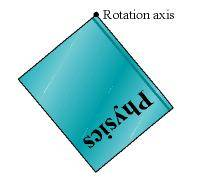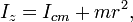# Book Pendulum Oscillation

jellyman
1. In the figure below, a book is suspended at one corner so that it can swing like a pendulum parallel to its plane. The edge lengths along the book face are 28 cm and 19 cm. If the angle through which it swings is only a few degrees, what is the period of the motion?2. I=(ML^2)/12
I=(ML^2)/3
T=2∏√I/M*G*dcom

3. I got dcom by using the distance formula and it's .1692 m
Then I tried to using both inertia equations and using length, width. Then I plugged in all the numbers. (multiple attempts)

All wrong answers (.788s, .394s, 1.062s)

.

Homework Helper
You don't have the right moments of inertia.

jellyman
Oh.

Then that leaves me with I= M(a2 + b2)/12.

That gave me a period of .476 second which has been marked wrong.

Homework Helper
Yep - that's the wrong moment of inertia as well.
That is for an oblong rotating about it's center.

You book is not rotating about it's center - otherwise it could not act as a pendulum.

Look up: parallel axis theorem.

jellymanI|| = $\frac{1}{12}$Mdcom2 + Mdcom2

This doesn't make sense though because it leaves me with an M on the top in the equation for T.

EDIT: I asked somewhere else and they used T = 2π√[L/g] and got .83 seconds. This doesn't sound quite right. It's too easy this way!

Last edited:
$$I_{CM}=\frac{M}{12}(a^2+b^2)$$
##r## is the distance from the corner to the center of the book; by pythagoras: $$r^2=\frac{a^2}{4}+\frac{b^2}{4}$$... therefore, by the parallel axis theorem: $$I=I_{CM}+Mr^2=\cdots$$... you finish up.